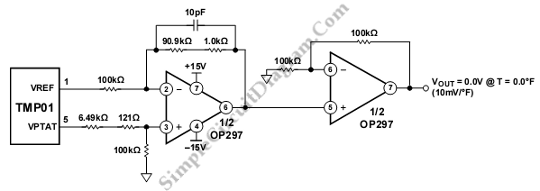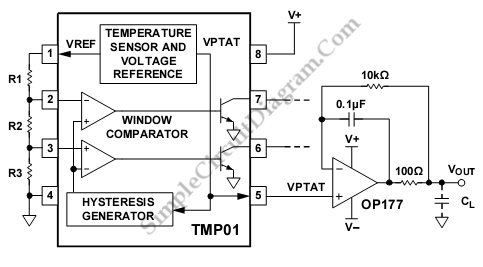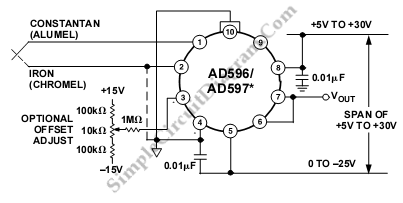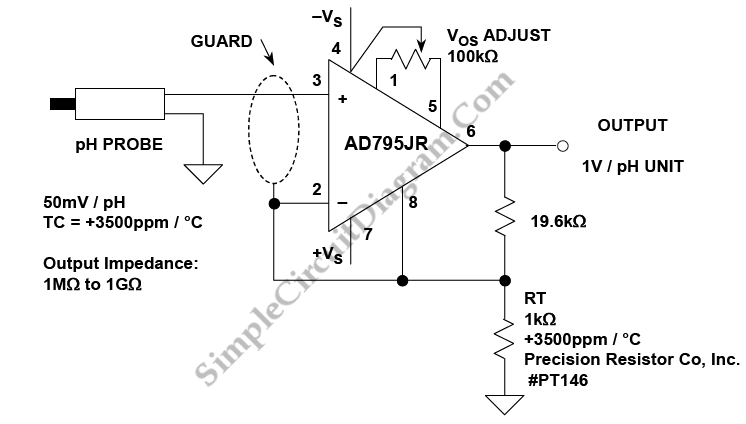TMP01 Fahrenheit Scale Temperature SensorThis schematic diagram is about TMP01 Fahrenheit Scale Temperature Sensor circuit. This circuit is used to convert VPTAT into an output that can be read directly in degrees Fahrenheit. The scale of this circuit is 10 mV/°F. This circuit consist of two stage,s first stage is differentiating circuit that has only unity gain or less. Then, the second stage is […]

Buffering TMP01 Temperature SensorThis is a buffer circuit for TMP01 Temperature Sensor. The output of this sensor is a low impedance dc output voltage with a 5 mV/K temperature coefficient. This sensor can be used in multiple control and measurement applications. The buffered VPTAT voltage output can drive 500 µA into 50 pF (maximum). Here is the schematic diagram of the circuit: To […]This is a AD596/AD597 J-K Thermocouple Signal Conditioner circuit. When operated with J and K type thermocouples, the AD596/AD597 can produce a temperature proportional output of 10 mV/°C. Thermocouples generate low level output voltages which are a function of both the reference or cold junction temperature and temperature being measured. The AD596/AD597 is used to compensate the cold junction temperature. […]